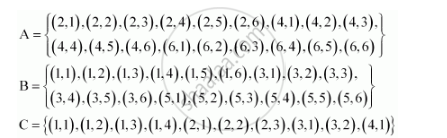CBSE (Arts) Class 11CBSE
Share

# Two Dice Are Thrown. the Events A, B and C Are as Follows: A and B Are Mutually Exclusive - CBSE (Arts) Class 11 - Mathematics

ConceptEvent Mutually Exclusive Events

#### Questions

Refer to question 6 above, state true or false: (give reason for your answer)
(i) A and B are mutually exclusive

Two Dice Are Thrown. the Events A, B and C Are as Follows: A and B Are Mutually Exclusive

#### Solution

Two dice are thrown. The events A, B and C are as follows:

A: getting an even number on the first die.

B: getting an odd number on the first die.

C: getting the sum of the numbers on the dice ≤ 5

A and B are mutually exclusiveIt is observed that A ∩ B = Φ

∴ A and B are mutually exclusive.

Thus, the given statement is true.

Is there an error in this question or solution?

#### Video TutorialsVIEW ALL 

Solution Two Dice Are Thrown. the Events A, B and C Are as Follows: A and B Are Mutually Exclusive Concept: Event - Mutually Exclusive Events.
S